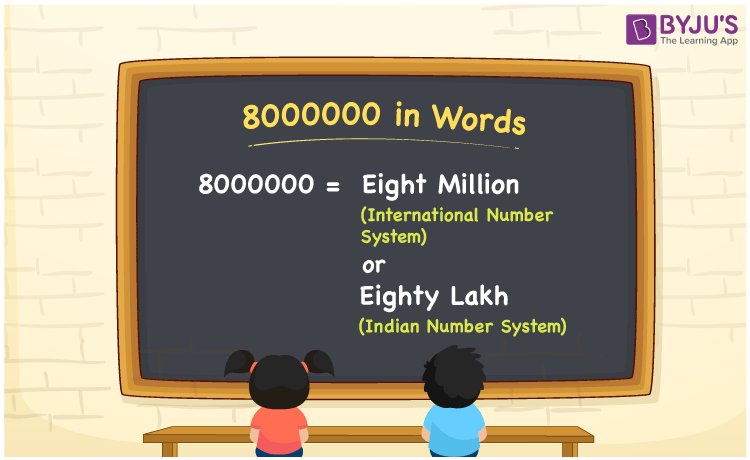# 8000000 in words

8000000 in words is written as Eighty Lakh in the Indian Numeral System and Eight Million in the International Numeral system. The article on Numeral System can give you an idea about the difference between both systems. The number 8000000 is used in expressions that relate to huge quantities, like money, population, area, social media views, distance between celestial bodies. If expressed in terms of social media views, we can say that “A new movie trailer is trending with Eight Million views.” In terms of population, we can use 8000000 as “The total population of Himachal Pradesh and Chandigarh is almost Eighty Lakh.”

Numeral System →

Indian Numeral System

International Numeral System

8000000 in words

Eighty Lakh

Eight Million

Eighty Lakh in Numbers

80,00,000

Eight Million in Numbers

8,000,000

## 8000000 in English Words## How to Write 8000000 in Words?

We can convert 8000000 to words using a place value chart. The number 8000000 has 7 digits, so let’s make a chart that shows the place value up to 7 digits. Different tables to show both Numeral Systems are given.

Indian System

 Ten Lakhs Lakhs Ten Thousands Thousands Hundreds Tens Ones 8 0 0 0 0 0 0

Thus, we can write the expanded form as:

8 × Ten Lakhs + 0 × Lakhs + 0 × Ten thousand + 0 × Thousand + 0 × Hundred + 0 × Ten + 0 × One

= 8 × 1000000 + 0 × 100000 + 0 × 10000 + 0 × 1000 + 0 × 100 + 0 × 10 + 0 × 1

= 8000000

= Eighty Lakh.

International System

 Million Hundred Thousands Ten Thousands Thousands Hundreds Tens Ones 8 0 0 0 0 0 0

Thus, we can write the expanded form as:

8 × Million + 0 × Hundred Thousand + 0 × Ten thousand + 0 × Thousand + 0 × Hundred + 0 × Ten + 0 × One

= 8 × 1000000 + 0 × 100000 + 0 × 10000 + 0 × 1000 + 0 × 100 + 0 × 10 + 0 × 1

= 8000000

= Eight Million.

8000000 is the natural number that is succeeded by 7999999 and preceded by 8000001.

8000000 in words – Eighty Lakh (Indian System) and Eight Million (International System).

Is 8000000 an odd number? – No.

Is 8000000 an even number? – Yes.

Is 8000000 a perfect square number? – No.

Is 8000000 a perfect cube number? – Yes. ( 200 × 200 × 200 )

Is 8000000 a prime number? – No.

Is 8000000 a composite number? – Yes.

## Solved Example

1. Write the number 8000000 in expanded form.

Solution: 8 × 1000000 + 0 × 100000 + 5 × 10000 + 0 × 1000 + 0 × 100 + 0 × 10 + 0 × 1

We can write 8000000 = 8000000 + 00000 + 0 + 0 + 0 + 0 + 0

Therefore it will be

= 8 × 1000000 + 0 × 100000 + 0 × 10000 + 0 × 1000 + 0 × 100 + 0 × 10 + 0 × 1

## Frequently Asked Questions on 8000000 in words

Q1

### How to write the number 8000000 in words?

8000000 in words is written as Eighty Lakh in the Indian System and Eight Million in the International System.
Q2

### 8000000 is a perfect cube number. True or False?

True. 8000000 is a perfect cube number. 200 × 200 × 200 is 8000000.
Q3

### Is 8000000 divisible by 10?

Yes. 8000000 is divisible by 10.